## Recommended PostsHello,

I have been trying to draw an arc with exact length and diamter but with no good result.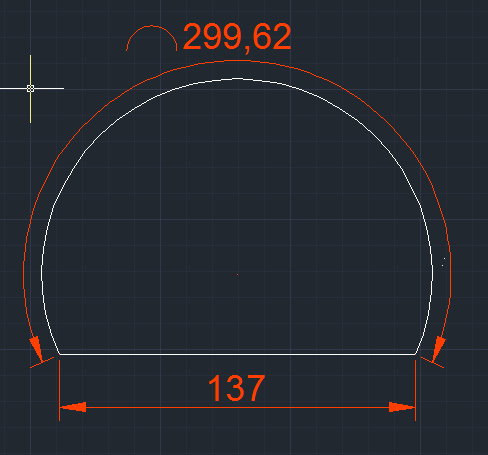You can see the image. Now, I want the diameter to be 137 mm and that was easy to drawTo draw an arc is not difficult BUT I can´t seem to find how/where to input the exact length that I want. In my case I want it to be 297 mm and NOT 299.62 as shown in the picture. Also ONE extra question: Why can´t I use Properties to change the length of this arc?

I hope someone will help me with this I guess easy problem.

Thanks in advance and best regards to you all,

Miro

##### Share on other sites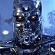Use the LENGTHEN command with the Total option.

##### Share on other sitesThe line that is dimensioned as 137mm is called a chord, NOT a diameter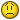##### Share on other sitesYou drew a chord of 137. Is that what you wanted, or did you want 137 for the diameter? You can change the radius in the Properties. I do not know how you might change the length via Properties, but you cannot hold the angle and the radius and yet change the length, thus AutoCAD would have to know or decide on its own what to hold if you have several editable interdependent parameters.

You can use the Lengthen command. Use the Total option, input the new length, then select the object, in this case an Arc. Try it. It shortens/lengthens the end you pick closest to.

##### Share on other sitesThank you for your quick replay. Yes I did mean chord and not diamter, my fault. And YES I want the chord to be 137 mm. I tried command LENGTHEN but I guess I must be doing something wrong. So here is how I do it.

I draw the line 137 mm, then I choose arc (3-point),select those 3 points and make the arc longer then 297 mm. So now I type LENGTHEN sa command, press T

write 297, select object (arc) and then I get a funny looking arc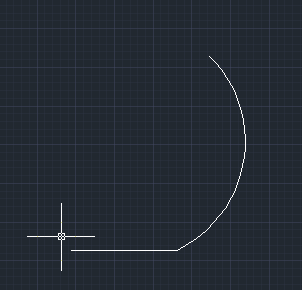Thaks for helping

##### Share on other sitesOne other option would be to use the MEASURE command to place a point on the arc. Then use the Break command. You'll be picking two points. The first will be the point (use the Node OSnap) and the second pick make off the end of the arc. The length of arc will change as will either the start or end angle but the radius will not change.Just when I come up with a second answer the OP changes the object he wants to edit. Go figure.##### Share on other sitesPerhaps this can not be easily drawn in AutoCAD. Try Googling for solutions and you could come up with this.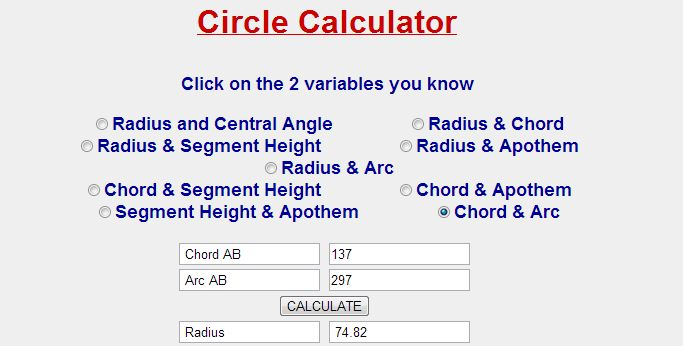##### Share on other sitesI guess you are right (http://www.1728.org/circsect.htm )

Thanks

##### Share on other sitesIn Autodesk Inventor this is rather trivial as Inventor as a Parametric Arc Length dimension tool.

You could easily set up Parameters in AutoCAD to do the same thing.

I will try to post an AutoCAD example later.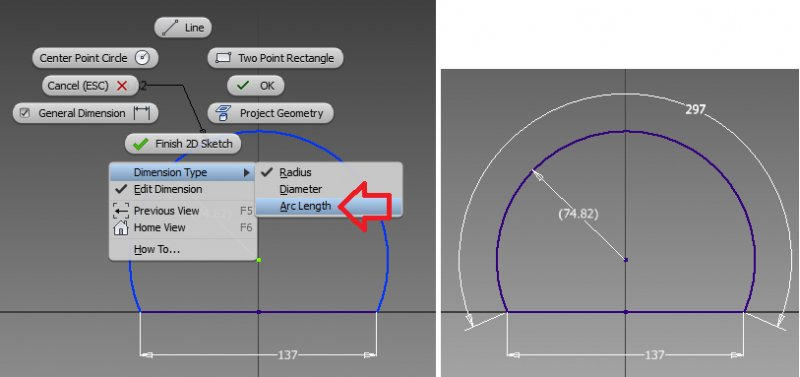The dimension in parenthesis means the radius is a driven dimension, or reference, not a driving dimension.

##### Share on other sitesa bit more reference information if you don't want to take the time to set up Parameters in AutoCAD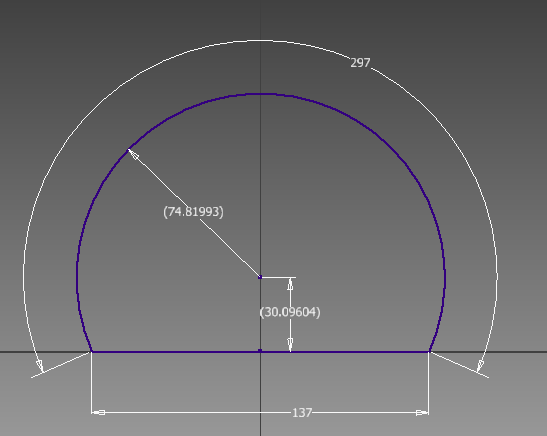##### Share on other sitesI didn't take the time to set up the full parametric relationship (so that any (valid) arc length can be specified).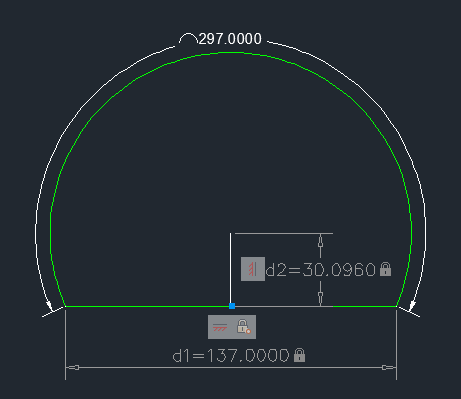##### Share on other sitesJust when I come up with a second answer the OP changes the object he wants to edit. Go figure.Yeah, man, I feel your pain.First time, huh? LOL. That would be some record if it were!

But the rest of the thread is proving of interest to me, as I've unsuccessfully dealt with the very option being offered here via Circle Calculator, etc.

##### Share on other sites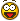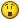Jesus...JD Mather...no comment...thanks,wow

##### Share on other sitesPerhaps this can not be easily drawn in AutoCAD. Try Googling for solutions and you could come up with this.

This is good, eldon. This has the Chord&Arc option I've been referring to in another thread. But now it looks like you're saying it's not so easy after all, even to draw.

##### Share on other sitesI didn't take the time to set up the full parametric relationship (so that any (valid) arc length can be specified).

JD, your Inventor solution looks great! I’m not sure how you got the arc to be 297 in AutoCAD, unless you were using results from Inventor, or from the Circle Calculator. I’ve not yet seen where you can constrain the length of the Arc to a user input value or a fixed one. In general, I’m not seeing how you constrained your figure to give the desired output without already knowing what it is supposed to be. If you could elaborate, that would be appreciated. Thanks in advance.

##### Share on other sitesIf you label the geometry in the following way: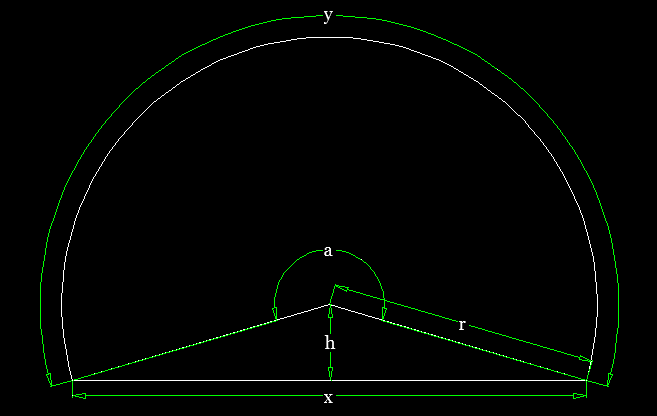Then

```   y = ar
a = (2π - 2tan^-1(x/2h))
=> y = (2π - 2tan^-1(x/2h))r```

And:

```   r^2 = (x/2)^2+h^2
=> r   = 1/2sqrt(x^2+4h^2)```

Therefore:

`y = sqrt(x^2+4h^2)(π-tan^-1(x/2h))`

If you can rearrange the above equation for h in terms of x & y, then you can solve the problem algebraically.

##### Share on other sitesIf you can rearrange the above equation for h in terms of x & y, then you can solve the problem algebraically.

Dang Lee, I was hoping you would to that last derivationMust involve some hyperbolic cosines and natural logs.

##### Share on other sitesI missed a trick!

After a bit of research, I discovered that equations of the form derived above cannot be solved algebraically, but you can use Newton's Method to solve the following relationship numerically:

`<chord-length>/<arc-length> = sin(x)/x`

This method is described here.

In code, this might be:

```;; Arc from Arc Length & Chord Length  -  Lee Mac
;; Uses Newton's Method to solve the relationship:
;; <chord-length>/<arc-length> = sin(x)/x

(defun c:arcl ( / a b c k p q r s x z )
(while
(and
(setq p (getpoint "\n1st point of Chord: "))
(setq q (getpoint "\n2nd point of Chord: " p))
(equal 0.0 (setq c (distance p q)) 1e-8)
)
(princ "\nPoints must be distinct.")
)
(if (and p q)
(progn
(princ (strcat "\nChord Length: " (rtos c)))
(while (and (setq s (getdist "\nArc Length: ")) (< s c))
(princ "\nArc Length must be greater than Chord Length.")
)
(if s
(progn
(setq p (trans p 1 0)
q (trans q 1 0)
k (/ c s)
x (sqrt (- 6 (* 6 k)))
)
(repeat 8
(setq x (- x (/ (- (sin x) (* k x)) (- (cos x) k))))
)
(setq r (/ s (* 2.0 x))
b (polar
(mapcar '(lambda ( a b ) (/ (+ a b) 2.0)) p q)
(+ (angle p q) (/ pi 2.0))
(* r (cos (/ (- (+ pi pi) x x) 2.0)))
)
)
(if (not (equal (* r (- (angle b q) (angle b p))) s 1e-8))
(setq z p p q q z)
)
(entmake
(list
'(0 . "ARC")
(cons 10 b)
(cons 40 r)
(cons 50 (angle b p))
(cons 51 (angle b q))
)
)
)
)
)
)
(princ)
)```

Quick Demo

Edited by Lee Mac

##### Share on other sites(not set to mm) Used your numbers

##### Share on other sitesAs far as I was aware the radius was an unknown parameter, else the construction would be impossible for an arbitrary radius, arc-length & chord-length.

## Join the conversation

You can post now and register later. If you have an account, sign in now to post with your account.
Note: Your post will require moderator approval before it will be visible.×   Pasted as rich text.   Paste as plain text instead

Only 75 emoji are allowed.